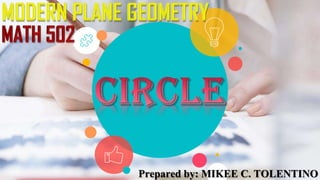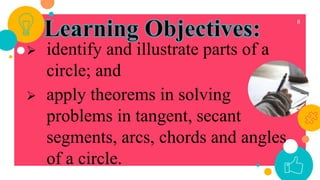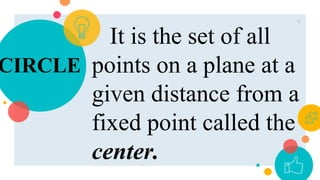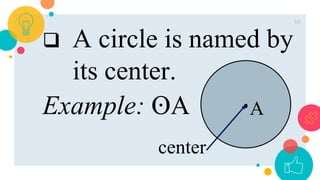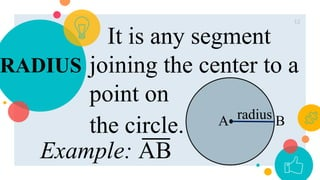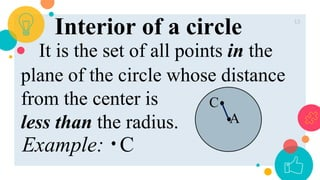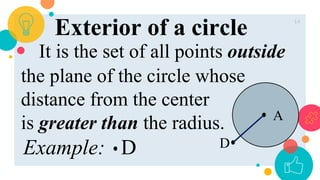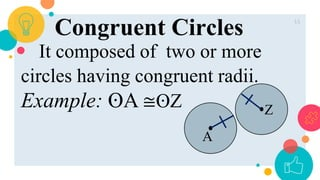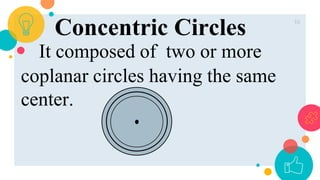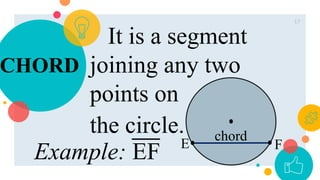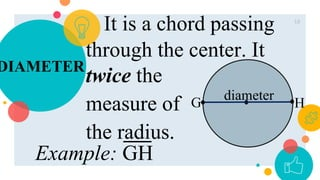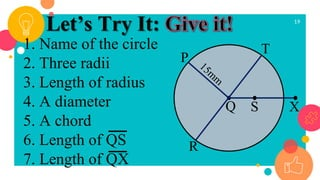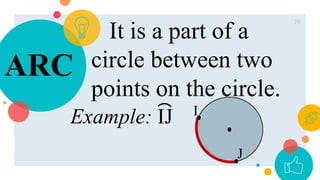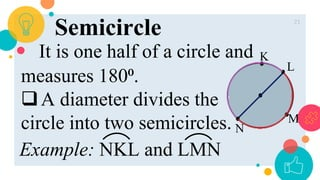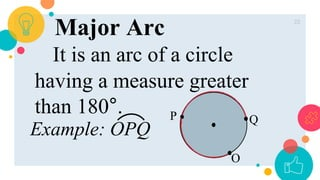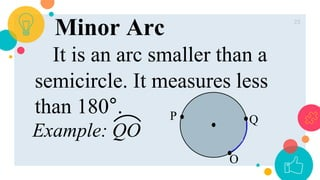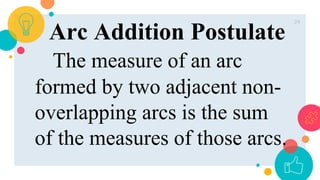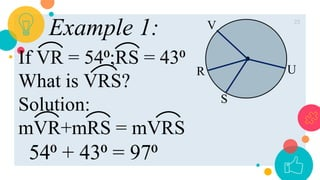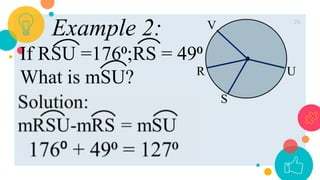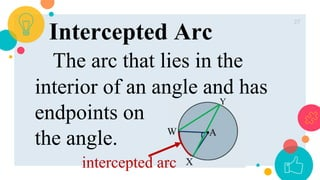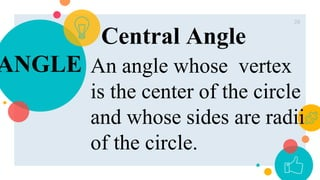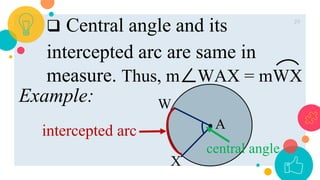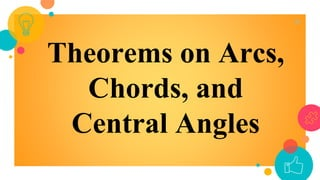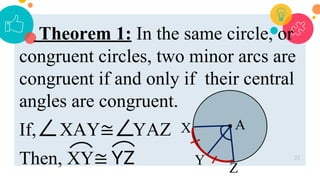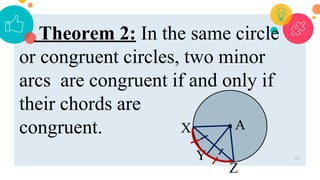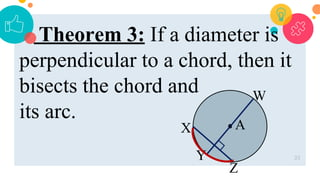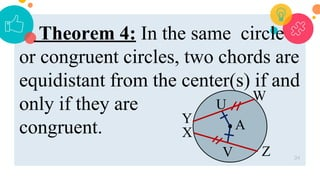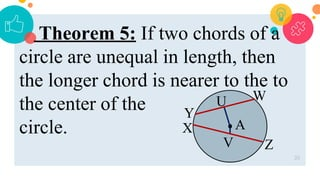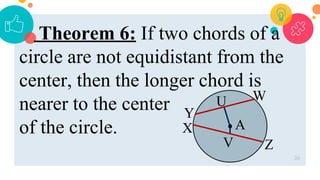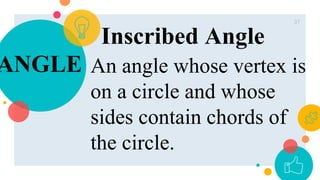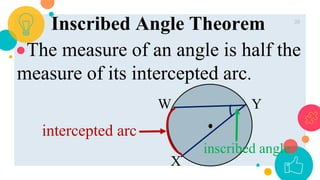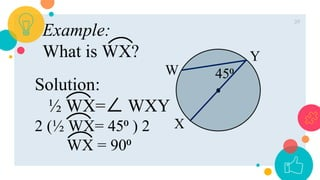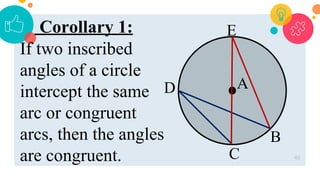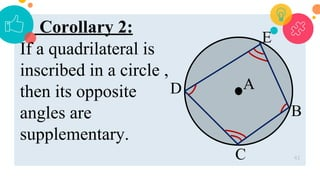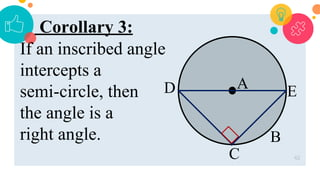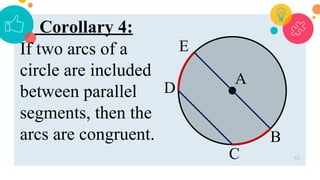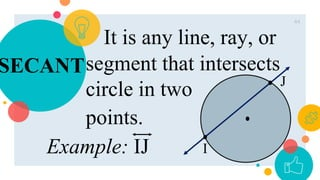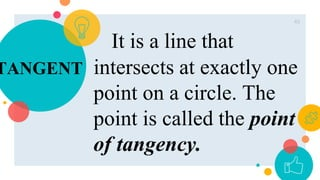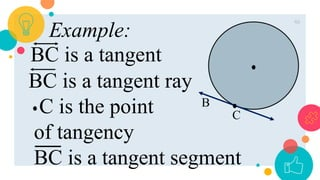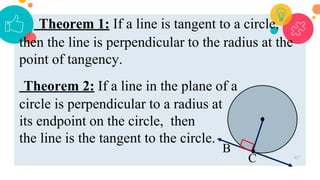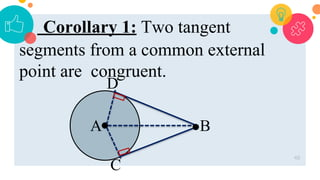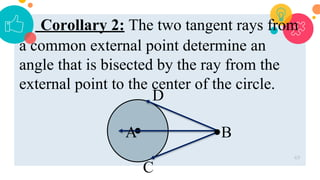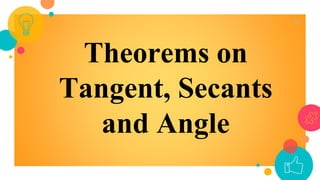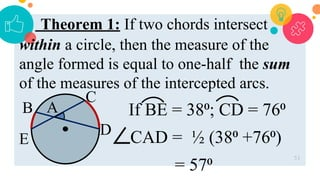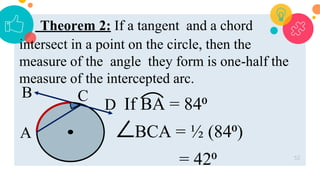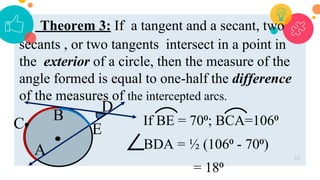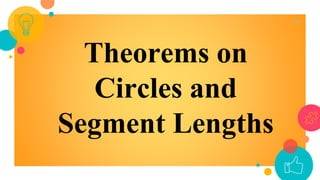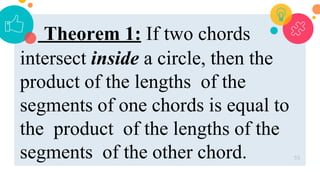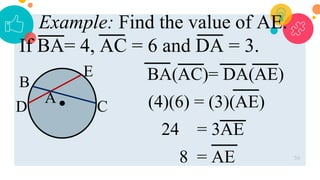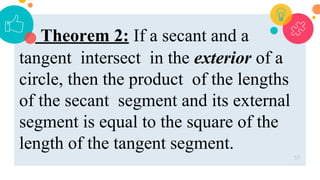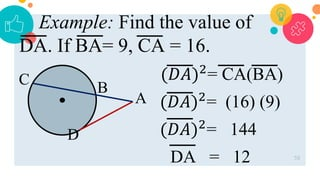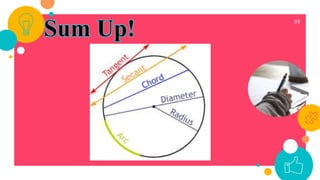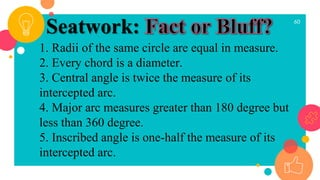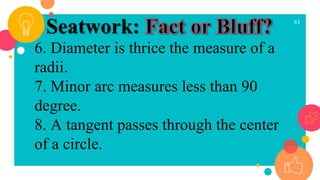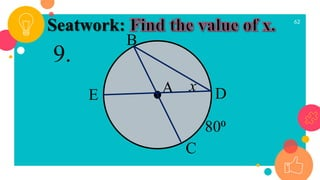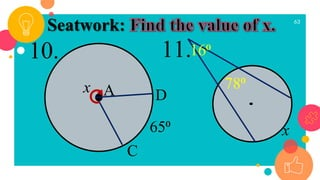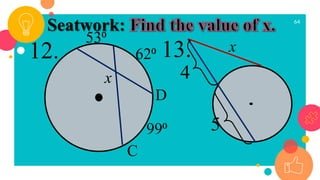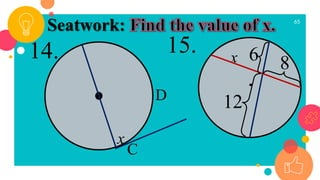1 of 67

## RecommendedModule 1 circlesdionesioable10.1 tangents to circlesamriawalludin2

### Math-502-Modern-Plane-Geometry-CIRCLE.pptx

1. 2 I understand
2. 3 H2O (Water)
3. 4 Apple Pie
4. 5 Copyright COPI COPPY COPY
5. 6 Summary mary + mary
6. Prepared by: MIKEE C. TOLENTINO
7. 8  identify and illustrate parts of a circle; and  apply theorems in solving problems in tangent, secant segments, arcs, chords and angles of a circle.
8. CIRCLE 9 It is the set of all points on a plane at a given distance from a fixed point called the center.
9. 10  A circle is named by its center. Example: ʘA A center
10. 11
11. RADIUS 12 It is any segment joining the center to a point on the circle. radius A B Example: AB
12. 13 Interior of a circle It is the set of all points in the plane of the circle whose distance from the center is less than the radius. A C Example: C
13. 14 Exterior of a circle It is the set of all points outside the plane of the circle whose distance from the center is greater than the radius. A D Example: D
14. 15 Congruent Circles It composed of two or more circles having congruent radii. Example: ʘA ≅ʘZ A Z
15. 16 Concentric Circles It composed of two or more coplanar circles having the same center.
16. CHORD 17 It is a segment joining any two points on the circle. chord E F Example: EF
17. DIAMETER 18 It is a chord passing through the center. It twice the measure of the radius. diameter H G Example: GH
18. 19 1. Name of the circle 2. Three radii 3. Length of radius 4. A diameter 5. A chord 6. Length of QS 7. Length of QX Let’s Try It: Q S X T R P
19. ARC 20 It is a part of a circle between two points on the circle. I J Example: IJ
20. 21 Semicircle It is one half of a circle and measures 180⁰. A diameter divides the circle into two semicircles. K L M N Example: NKL and LMN
21. 22 Major Arc It is an arc of a circle having a measure greater than 180°. Example: OPQ O P Q
22. 23 Minor Arc It is an arc smaller than a semicircle. It measures less than 180°. Example: QO O P Q
23. 24 Arc Addition Postulate The measure of an arc formed by two adjacent non- overlapping arcs is the sum of the measures of those arcs.
24. 25 Example 1: If VR = 54⁰;RS = 43⁰ What is VRS? Solution: mVR+mRS = mVRS 54⁰ + 43⁰ = 97⁰ R S U V
25. 26 Example 2: If RSU =176⁰;RS = 49⁰ What is mSU? R S U V
26. 27 Intercepted Arc The arc that lies in the interior of an angle and has endpoints on the angle. Y intercepted arc
27. ANGLE 28 Central Angle An angle whose vertex is the center of the circle and whose sides are radii of the circle.
28. 29 Example:  Central angle and its intercepted arc are same in measure. Thus, m WAX = mWX central angle intercepted arc A W X
29. Theorems on Arcs, Chords, and Central Angles 30
30. Theorem 1: In the same circle, or congruent circles, two minor arcs are congruent if and only if their central angles are congruent. If, XAY≅ YAZ Then, XY≅ YZ 31 A X Y Z
31. Theorem 2: In the same circle or congruent circles, two minor arcs are congruent if and only if their chords are congruent. 32 A X Y Z
32. Theorem 3: If a diameter is perpendicular to a chord, then it bisects the chord and its arc. 33 A X Y Z W
33. Theorem 4: In the same circle or congruent circles, two chords are equidistant from the center(s) if and only if they are congruent. 34 A X Y Z W U V
34. Theorem 5: If two chords of a circle are unequal in length, then the longer chord is nearer to the to the center of the circle. 35 A X Y Z W U V
35. Theorem 6: If two chords of a circle are not equidistant from the center, then the longer chord is nearer to the center of the circle. 36 A X Y Z W U V
36. ANGLE 37 Inscribed Angle An angle whose vertex is on a circle and whose sides contain chords of the circle.
37. 38 Inscribed Angle Theorem The measure of an angle is half the measure of its intercepted arc. inscribed angle intercepted arc Y W X
38. 39 Solution: ½ WX= WXY 2 (½ WX= 45⁰ ) 2 WX = 90⁰ Y W X 45⁰ Example: What is WX?
39. Corollary 1: If two inscribed angles of a circle intercept the same arc or congruent arcs, then the angles are congruent. 40 C D B A E
40. C D B A Corollary 2: If a quadrilateral is inscribed in a circle , then its opposite angles are supplementary. 41 E
41. Corollary 3: If an inscribed angle intercepts a semi-circle, then the angle is a right angle. 42 C D B A E
42. Corollary 4: If two arcs of a circle are included between parallel segments, then the arcs are congruent. 43 E C D B A
43. SECANT 44 It is any line, ray, or segment that intersects circle in two points. J I Example: IJ
44. TANGENT 45 It is a line that intersects at exactly one point on a circle. The point is called the point of tangency.
45. 46 Example: BC is a tangent BC is a tangent ray C is the point of tangency BC is a tangent segment B C
46. Theorem 1: If a line is tangent to a circle, then the line is perpendicular to the radius at the point of tangency. Theorem 2: If a line in the plane of a circle is perpendicular to a radius at its endpoint on the circle, then the line is the tangent to the circle. 47 B C
47. Corollary 1: Two tangent segments from a common external point are congruent. 48 A C B D
48. Corollary 2: The two tangent rays from a common external point determine an angle that is bisected by the ray from the external point to the center of the circle. 49 A C B D
49. Theorems on Tangent, Secants and Angle 50
50. Theorem 1: If two chords intersect within a circle, then the measure of the angle formed is equal to one-half the sum of the measures of the intercepted arcs. If BE = 38⁰; CD = 76⁰ CAD = ½ (38⁰ +76⁰) = 57⁰ 51 B A C D E
51. Theorem 2: If a tangent and a chord intersect in a point on the circle, then the measure of the angle they form is one-half the measure of the intercepted arc. If BA = 84⁰ BCA = ½ (84⁰) = 42⁰ 52 B A C D
52. Theorem 3: If a tangent and a secant, two secants , or two tangents intersect in a point in the exterior of a circle, then the measure of the angle formed is equal to one-half the difference of the measures of the intercepted arcs. If BE = 70⁰; BCA=106⁰ BDA = ½ (106⁰ - 70⁰) = 18⁰ 53 B A C D E
53. Theorems on Circles and Segment Lengths 54
54. Theorem 1: If two chords intersect inside a circle, then the product of the lengths of the segments of one chords is equal to the product of the lengths of the segments of the other chord. 55
55. Example: Find the value of AE. If BA= 4, AC = 6 and DA = 3. BA(AC)= DA(AE) (4)(6) = (3)(AE) 24 = 3AE 8 = AE 56 B A C D E
56. Theorem 2: If a secant and a tangent intersect in the exterior of a circle, then the product of the lengths of the secant segment and its external segment is equal to the square of the length of the tangent segment. 57
57. Example: Find the value of DA. If BA= 9, CA = 16. (𝐷𝐴)2 = CA(BA) (𝐷𝐴)2 = (16) (9) (𝐷𝐴)2 = 144 DA = 12 58 B A C D
58. 59
59. 60 1. Radii of the same circle are equal in measure. 2. Every chord is a diameter. 3. Central angle is twice the measure of its intercepted arc. 4. Major arc measures greater than 180 degree but less than 360 degree. 5. Inscribed angle is one-half the measure of its intercepted arc. Seatwork:
60. 61 6. Diameter is thrice the measure of a radii. 7. Minor arc measures less than 90 degree. 8. A tangent passes through the center of a circle. Seatwork:
61. 62 Seatwork: C x B A 80⁰ D 9. E
62. 63 Seatwork: C x A 65⁰ D 10. 11. x 16⁰ 78⁰
63. 64 Seatwork: C 53⁰ D 12. 13. x 4 5 x 99⁰ 62⁰
64. 65 Seatwork: C D 14. 15. x 6 12 x 8
65. “ 66
66. 67